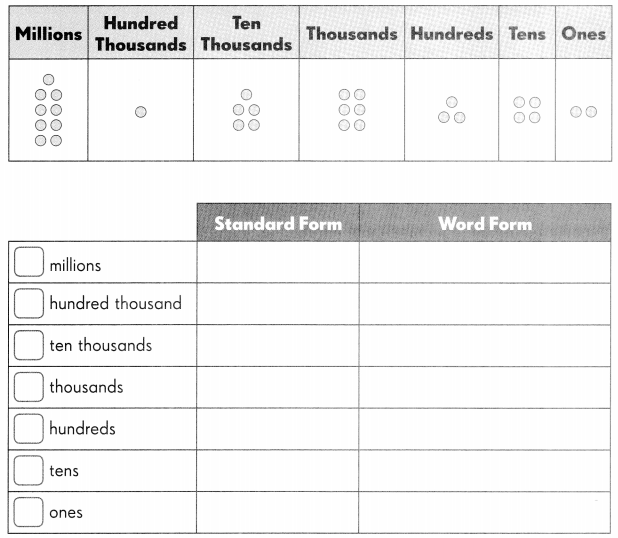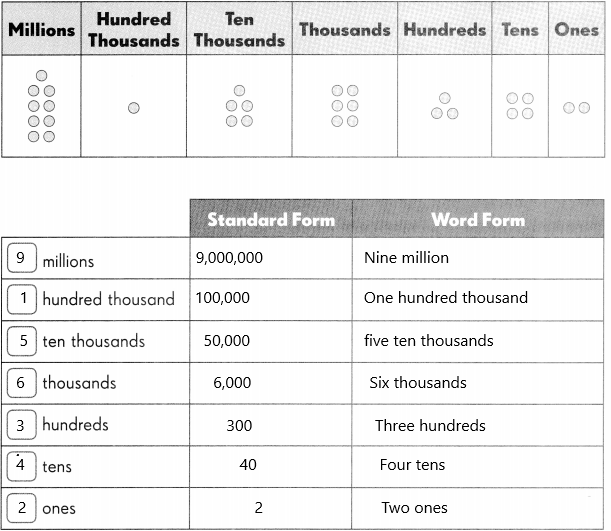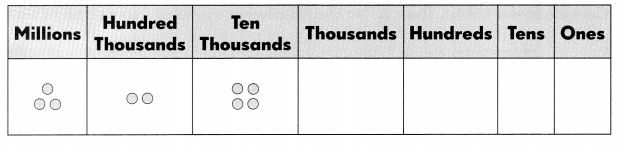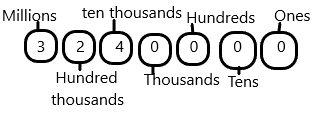# Math in Focus Grade 5 Chapter 1 Practice 2 Answer Key Numbers to 10,000,000

Go through the Math in Focus Grade 5 Workbook Answer Key Chapter 1 Practice 2 Numbers to 10,000,000 to finish your assignments.

## Math in Focus Grade 5 Chapter 1 Practice 2 Answer Key Numbers to 10,000,000

Complete the table. Then write the number in standard form and in word form.

Question 1.Number in standard form: ______
Number in word form: _____
Place value in Maths describes the position or place of a digit in a number. Each digit has a place in a number. When we represent the number in general form, the position of each digit will be expanded. Those positions start from a unit place or we also call it one’s position. The order of place value of digits of a number of right to left is units, tens, hundreds, thousands, ten thousand, a hundred thousand, and so on.
1. Place value tells you how much each digit stands for
2. Use a hyphen when you use words to write 2-digit numbers greater than 20 that have a digit other than zero in the one’s place.
3. A place-value chart tells you how many hundreds, tens, and ones to use.Number in standard form: 9,156,342
Number in word form: Nine million, one hundred fifty-six thousand, three hundred forty-two.

Write the number in standard form and in word form.Number in standard form: __________________
Number in word form: _____________________
Place value in Maths describes the position or place of a digit in a number. Each digit has a place in a number. When we represent the number in general form, the position of each digit will be expanded. Those positions start from a unit place or we also call it one’s position. The order of place value of digits of a number of right to left is units, tens, hundreds, thousands, ten thousand, a hundred thousand, and so on.
1. Place value tells you how much each digit stands for
2. Use a hyphen when you use words to write 2-digit numbers greater than 20 that have a digit other than zero in the one’s place.
3. A place-value chart tells you how many hundreds, tens, and ones to use.Number in standard form:3,240,000.
Number in word form: Three million two hundred forty thousand.

Write each number in standard form.

Question 3.
two million, one hundred fifty-six thousand, four _____
Standard form definition: A standard form of a number in Maths is basically mentioned for the representation of large numbers or small numbers. We use exponents to represent such numbers in standard form.
How to write the standard form of a number:
The steps to write the standard form of a number are as follows:
Step 1: Write the first number from the given number.
Step 2: Add the decimal point after the first number.
Step 3: Now, count the number of digits after the first number from the given number and write it in the power of 10.
The number is 2,156,004. Thus the standard form of a number is obtained as follows:
Step 1: The first number is 2.
Step 2: Adding the decimal point after 2, “it becomes 2.”
Step 3: The number of digits after 2 is 6.
Hence, the standard form of 2,156,004 is 2 × 106.

Question 4.
five million, two hundred thirty-eight thousand _____
Standard form definition: A standard form of a number in Maths is basically mentioned for the representation of large numbers or small numbers. We use exponents to represent such numbers in standard form.
How to write the standard form of a number:
The steps to write the standard form of a number are as follows:
Step 1: Write the first number from the given number.
Step 2: Add the decimal point after the first number.
Step 3: Now, count the number of digits after the first number from the given number and write it in the power of 10.
The number is 5,230,000. Thus the standard form of a number is obtained as follows:
Step 1: The first number is 5.
Step 2: Adding the decimal point after 5, “it becomes 5.”
Step 3: The number of digits after 5 is 6.
Hence, the standard form of 5,230,000 is 2 × 106.

Question 5.
seven million, one hundred fifty thousand _____
Standard form definition: A standard form of a number in Maths is basically mentioned for the representation of large numbers or small numbers. We use exponents to represent such numbers in standard form.
How to write the standard form of a number:
The steps to write the standard form of a number are as follows:
Step 1: Write the first number from the given number.
Step 2: Add the decimal point after the first number.
Step 3: Now, count the number of digits after the first number from the given number and write it in the power of 10.
The number is 7,150,000. Thus the standard form of a number is obtained as follows:
Step 1: The first number is 7.
Step 2: Adding the decimal point after 7, “it becomes 7.”
Step 3: The number of digits after 7 is 6.
Hence, the standard form of 7,150,000 is 2 × 106.

Question 6.
six million, sixty thousand, fifty _____
Standard form definition: A standard form of a number in Maths is basically mentioned for the representation of large numbers or small numbers. We use exponents to represent such numbers in standard form.
How to write the standard form of a number:
The steps to write the standard form of a number are as follows:
Step 1: Write the first number from the given number.
Step 2: Add the decimal point after the first number.
Step 3: Now, count the number of digits after the first number from the given number and write it in the power of 10.
The number is 6,060,050. Thus the standard form of a number is obtained as follows:
Step 1: The first number is 6.
Step 2: Adding the decimal point after 6, “it becomes 6.”
Step 3: The number of digits after 6 is 6.
Hence, the standard form of 6,060,050 is 2 × 106.

Question 7.
three million, three ____
Standard form definition: A standard form of a number in Maths is basically mentioned for the representation of large numbers or small numbers. We use exponents to represent such numbers in standard form.
How to write the standard form of a number:
The steps to write the standard form of a number are as follows:
Step 1: Write the first number from the given number.
Step 2: Add the decimal point after the first number.
Step 3: Now, count the number of digits after the first number from the given number and write it in the power of 10.
The number is 3,000,003. Thus the standard form of a number is obtained as follows:
Step 1: The first number is 3.
Step 2: Adding the decimal point after 3, “it becomes 3.”
Step 3: The number of digits after 3 is 6.
Hence, the standard form of 3,000,003 is 2 × 106.

Write each number in word form.

Question 8.
5,050000 _____
Numbers in words are written using the English alphabet. Numbers can be expressed both in words and figures. For example, 100,000 in words is written as One Lakh or One hundred thousand. Numbers in words can be written for all the natural numbers, based on the place value of digits, such as ones, tens, hundreds, thousands, and so on.
How to write numbers in words:
The rules to write the numbers in words are discussed here. To write any number in the form of words, we have to check the place value. Let us learn this by observing the below steps.
1. The place value of one’s, a number from 1 to 9 is written in words as, one, two, three, four, five, six, seven, eight and nine.
2. The least number which is at tens place is 10, which is written in words as ten.
3. The numbers from 11 to 19 are written in words as, eleven, twelve, thirteen, fourteen, fifteen, sixteen, seventeen, eighteen and nineteen. And the next number is twenty.
4. After twenty, the numbers follow the pattern in such a way that they are written in words as thirty, forty, fifty, sixty, seventy, eighty, ninety and so on.
5. The preceding numbers are linked with these words and mentioned from one to nine. For example, twenty-one, twenty-two, etc.
If we have to write 5,050,000 in words, then it will be written as:
Number in word form: Five million fifty thousand.

Question 9.
8,147,600 ____
Numbers in words are written using the English alphabet. Numbers can be expressed both in words and figures. For example, 100,000 in words is written as One Lakh or One hundred thousand. Numbers in words can be written for all the natural numbers, based on the place value of digits, such as ones, tens, hundreds, thousands, and so on.
How to write numbers in words:
The rules to write the numbers in words are discussed here. To write any number in the form of words, we have to check the place value. Let us learn this by observing the below steps.
1. The place value of one’s, a number from 1 to 9 is written in words as, one, two, three, four, five, six, seven, eight and nine.
2. The least number which is at tens place is 10, which is written in words as ten.
3. The numbers from 11 to 19 are written in words as, eleven, twelve, thirteen, fourteen, fifteen, sixteen, seventeen, eighteen and nineteen. And the next number is twenty.
4. After twenty, the numbers follow the pattern in such a way that they are written in words as thirty, forty, fifty, sixty, seventy, eighty, ninety and so on.
5. The preceding numbers are linked with these words and mentioned from one to nine. For example, twenty-one, twenty-two, etc.
If we have to write 8,147,600 in words, then it will be written as:
Number in word form: Eight million one hundred forty-seven thousand six hundred.

Question 10.
7,230,014 ____
Numbers in words are written using the English alphabet. Numbers can be expressed both in words and figures. For example, 100,000 in words is written as One Lakh or One hundred thousand. Numbers in words can be written for all the natural numbers, based on the place value of digits, such as ones, tens, hundreds, thousands, and so on.
How to write numbers in words:
The rules to write the numbers in words are discussed here. To write any number in the form of words, we have to check the place value. Let us learn this by observing the below steps.
1. The place value of one’s, a number from 1 to 9 is written in words as, one, two, three, four, five, six, seven, eight and nine.
2. The least number which is at tens place is 10, which is written in words as ten.
3. The numbers from 11 to 19 are written in words as, eleven, twelve, thirteen, fourteen, fifteen, sixteen, seventeen, eighteen and nineteen. And the next number is twenty.
4. After twenty, the numbers follow the pattern in such a way that they are written in words as thirty, forty, fifty, sixty, seventy, eighty, ninety and so on.
5. The preceding numbers are linked with these words and mentioned from one to nine. For example, twenty-one, twenty-two, etc.
If we have to write 7,230,014 in words, then it will be written as:
Number in word form: Seven million two hundred thirty thousand fourteen.

Question 11.
5,192,622 ____
Numbers in words are written using the English alphabet. Numbers can be expressed both in words and figures. For example, 100,000 in words is written as One Lakh or One hundred thousand. Numbers in words can be written for all the natural numbers, based on the place value of digits, such as ones, tens, hundreds, thousands, and so on.
How to write numbers in words:
The rules to write the numbers in words are discussed here. To write any number in the form of words, we have to check the place value. Let us learn this by observing the below steps.
1. The place value of one’s, a number from 1 to 9 is written in words as, one, two, three, four, five, six, seven, eight and nine.
2. The least number which is at tens place is 10, which is written in words as ten.
3. The numbers from 11 to 19 are written in words as, eleven, twelve, thirteen, fourteen, fifteen, sixteen, seventeen, eighteen and nineteen. And the next number is twenty.
4. After twenty, the numbers follow the pattern in such a way that they are written in words as thirty, forty, fifty, sixty, seventy, eighty, ninety and so on.
5. The preceding numbers are linked with these words and mentioned from one to nine. For example, twenty-one, twenty-two, etc.
If we have to write 5,192,622 in words, then it will be written as:
Number in word form: Five million one hundred ninety-two thousand six hundred twenty-two.

Question 12.
9009,009 ____